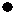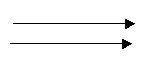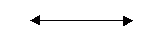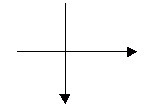Email us to get an instant 20% discount on highly effective K-12 Math & English kwizNET Programs!

#### Online Quiz (WorksheetABCD)

Questions Per Quiz = 2 4 6 8 10

### Grade 2 - Mathematics6.4 Points, Lines, and Segments

 Point: A point is a dot. Straight line: A line that has no end points, can be extended continuously on either sides. Segment: A line that has two end points is called a segment. Ray: A line that has only one end point is called ray. Parallel Lines : Two lines that do not touch each other are called parallel lines. Intersecting lines: Two lines that pass through each other are called intersecting lines. Perpendicular lines: Two lines that form a L shape are called perpendicular lines. Directions: Answer the following questions. Also draw point, lines (parallel lines, perpendicular and intersecting lines) and segment.
 Q 1: In math a point is an exact spot. You show a point with adotsemicoloncomma Q 2: If lines run side by side and never meet they are calledparallel linesintersecting linesperpendicular lines Q 3: These lines are _______ linesperpendicularhorizontalverticalparallel Q 4: Parallel lines always stay the same distance aparttruefalse Q 5: This figure is a ______lineparallelverticalhorizontalperpendicular Q 6: These lines are _______ linesverticalperpendicularhorizontalparallel Q 7: This figure is a ______linehorizontalparallelperpendicularvertical Q 8: If you put two points on a paper and connect them you will have apointcircleline segment Question 9: This question is available to subscribers only! Question 10: This question is available to subscribers only!